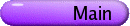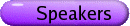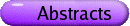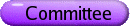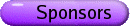Knot Homologies Org: Dror Bar-Natan (Toronto)[PDF] CARMEN CAPRAU, The University of Iowa, Iowa City, Iowa 52242 A sl(2) tangle homology for dotted, seamed cobordisms [PDF] We construct a sl(2) Bar-Natan like tangle homology for dotted, seamed cobordisms with Z[a] coefficients. This theory is functorial under link cobordisms. A version of the original Khovanov Homology corresponds to the choice a=0. Likewise, for a=-1 we recover Lee's theory. OLIVIER COLLIN, Université du Québec à Montréal Non-trivial actions on Floer homology [PDF] Given a finite order orientation-preserving diffeomorphism t: Y3 ® Y3 of an integer homology sphere Y3, it is an interesting and difficult general problem to understand the effect of the induced map on instanton Floer homology. In this talk, we consider odd-order diffeomorphisms of integer homology sphere and their induced effect on SU(2)-character varieties and Floer homology. We are interested in cases where the resulting equivariant Casson invariant differs from the Casson and the consequences for finding non-trivial actions on Floer homology. This provides the first examples of non-trivial odd-order actions on the Floer homology of irreducible homology spheres. We shall also see how this can be related to the existence of incompressible surfaces in the 3-manifold Y3. JOEL KAMNITZER, UC Berkeley, Berkeley, CA Knot homology via derived categories of coherent sheaves [PDF] We will give a construction of a knot homology theory using the derived category of coherent sheaves on a certain variety arising in geometric representation theory. MIKHAIL KHOVANOV, Columbia University Braid cobordisms and triangulated categories [PDF] We review known actions of the braid group on triangulated categories and their extensions to representations of the category of braid cobordisms. PETER LEE, University of Toronto, 40 St. George St., Toronto, Ontario, Canada Closed-Form Associators in a "Toy" Quotient [PDF] In the theory of finite-type invariants of knots, a key objective is to construct a "universal finite-type invariant", which takes values in a certain target space of chord diagrams. Construction of such a universal invariant can typically be reduced to determining its value on a particular kind of tangle, which represents a sort of "associativity" property. This value is usually referred to as an "associator", and it is hoped that finding an explicit expression for such an associator could offer significant knot-theoretic insights. In general, however, finding associators has proved to be an extremely difficult problem. In this talk I will discuss how a closed-form associator can be constructed in a particular "toy" quotient of the usual target space, which may ultimately help us to construct associators in more general target spaces. ROBERT LIPSHITZ, Columbia University, 2990 Broadway, New York, NY 10027, USA Some recent developments in Heegaard-Floer homology [PDF] We will discuss some recent developments in Heegaard-Floer homology-progress on making it combinatorial and attempts to generalize it. GAD NAOT, University of Toronto, Canada The Universal Khovanov Link Homology Theory-Extracting Algebraic Information [PDF] In this talk I will present the universal Khovanov link homology theory (n=2). This theory is developed using the full strength of the geometric formalism of Khovanov link homology theory and has many computational and theoretical advantages. The universal theory answers questions regarding the amount of algebraic information held within the complex associated to a link. It also answers questions regarding the extraction of this information by giving control over the various TQFTs applied to the complex (along with control over other gadgets such as the various spectral sequences related to these TQFTs). After a brief overview and some reminders I will introduce the major tools and ideas used in developing the universal theory (such as surface classification, genus generating operators, complex isomorphisms and "promotions"). Then, I will present some of the advantages of such a theory, time permitting (more on the topic can be found at arXiv:GT/0603347). JUAN ARIEL ORTIZ-NAVARRO, University of Iowa, Iowa City, IA Khovanov Homology and Reidemeister Torsion [PDF] The Reidemeister Torsion construction can be applied to the chain complex that is used to compute the Khovanov homology of a knot or a link. This defines a volume form on Khovanov homology. The volume form transforms correctly under Reidemeister moves to give an invariant volume on the Khovanov homology. We use this to study the invariants of knots. JAKE RASMUSSEN, Princeton University, Princeton, NJ, USA Stable KR-homology of torus knots [PDF] Computer calculations suggest that the limiting behavior of the Khovanov homology of T(m,n) as n ® ¥ is rather complicated. In contrast, the corresponding limit for the HOMFLY homology of T(m,n) is quite simple. I'll describe how to calculate this limit and explain why the result provides evidence for the presence of a symmetry in the HOMFLY homology. LEV ROZANSKY, University of North Carolina at Chapel Hill Virtual knots, convolutions and a categorification of the SO(2N) Kauffman polynomial [PDF] We present a categorification construction for the SO(2N) specialization of the Kauffman polynomial and prove its invariance under the first and second Reidemeister moves. The construction follows the Kauffman-Vogel alternating sign formula, which expresses the Kauffman polynomial of a link in terms of polynomials of 4-valent planar graphs. We define the matrix factorization associated to the 4-vertex as a convolution of a chain of two saddle morphisms, relating parallel and virtually crossing pairs of arcs. This is a joint work with M. Khovanov. PAUL SEIDEL, MIT, Room 2-270, 77 Massachusetts Ave., Cambridge, MA 02139 Localization in Floer homology and applications [PDF] I will explain how to construct localization maps for Z/2-actions in Floer theory, and how this explains the relation between the symplectic version of Khovanov homology and Ozsvath-Szabo theory. This is joint work with Ivan Smith. DYLAN THURSTON, Columbia ROBB TODD, University of Iowa Khovanov Homology and the Twist Number of Alternatinng Knots [PDF] O. Dasbach and X. S. Lin showed that the sum of the absolute value of the second and penultimate coefficient of the Jones polynomial of an alternating knot is equal to the twist number of the knot. Here we give a new proof of their result using a variant of Khovanov's homology that was defined by O. Viro for the Kauffman bracket. The proof is by induction on the number of crossings using the long exact sequence in Khovanov homology corresponding to the Kauffman bracket skein relation.